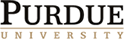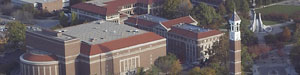Fall 2011
Time and place: Thursdays 3:30-4:30 in MATH 731
Organizer: Ralph Kaufmann. Contact organizer.

Abstracts

09/08/11 Lizhen Qin (Purdue). On Moduli Spacnes and CW Structures from Morse Theory
Abstract:
This talk will focus on various results concerning the moduli spaces of broken flow lines associated with a Morse function. We study the compactness of flow lines, manifold structures of certain compactified moduli spaces, orientation formulas, and CW structures on the underlying manifold.

09/15/11 Ben Ward (Purdue). Cyclic $A_\infty$ Algebras and Deligne's Conjecture
Abstract:
First we describe a class of homotopy Frobenius algebras via cyclic operads which we call cyclic $A_\infty$ algebras.  We then define a suitable new combinatorial operad which acts on the Hochschild cochains of such an algebra in a manner which encodes the homotopy BV structure.  Moreover we show that this operad is equivalent to the cellular chains of a certain topological (quasi)-operad of CW complexes whose constituent spaces form a homotopy associative version of the Cacti operad of Voronov.  These cellular chains thus constitute a chain model for the framed little disks operad, proving a cyclic $A_\infty$ version of Deligne's conjecture.

09/22/11 Ben McReynolds (Purdue). Geometric variants of topological bound problems
Abstract: In 1982, Hamrick and Royster proved that every compact flat n-manifold (topologically) bounds. Motivated by work of Gromov, Farrell and Zdravkovska conjectured that one can solve this bounding problem with a manifold that admits a complete negatively curved metric in it's interior. One candidate class of manifolds are finite volume, noncompact hyperbolic n-manifolds. These manifolds have cusp ends that are topologically flat (n-1)-manifolds and so produce examples of manifolds with flat boundaries. I will discuss my work on the geometric classification of these ends. The main technical tools employed are results that resolve various types of singularities under passage to some finite degree covering space.

10/06/11 Justin Young (IU). Brace Bar-Cobar Duality
Abstract:
After providing motivation for studying the E_2 algebra structure on the cochain complex of a space, we will consider the category of dg E_2 algebras. We will show that the classical bar-cobar duality between dg E_1 algebras and dg coalgebras can be enhanced to a duality between dg E_2 algebras and dg Hopf algebras. Over a field, this induces an isomorphism of homotopy categories between E_2 algebras and Hopf algebras. If time permits, I will discuss work in progress attempting to apply this duality to prove a conjecture of Mandell that with connectivity and dimension restrictions, and after inverting sufficiently many primes, the cochain complex of a space is equivalent as an E_2 algebra to a commutative algebra.

10/20/11 Jim McClure (Purdue). Verdier Duality and the Cap Product
Abstract:
It is well-known that there are two ways to prove Poincare duality,by using Verdier duality or by using the cap product.  In this talk we show that the Poincare duality isomorphisms obtained by these two methods are the same.  As a byproduct we show that the proof using Verdier duality can be done more simply without Verdier duality.

11/03/11 Angelica Osorno (U of Chicago). 2-vector spaces, 2-representations and 2-characters
Abstract:  In this talk we will describe an attempt to categorify certain notions in linear algebra and representation theory. We will define the notions of 2-vector space and 2-representation of a group. We will then define the 2-character of a 2-representation, which is a function on commuting pairs of elements in G, and derive expressions for the 2-character of a direct sum, tensor product, and induced 2-representations.
We will then establish a connection with the character theory developed by Hopkins, Kuhn and Ravenel, and state some conjectures of how this connection can be made more concrete.

Cancelled. Peter Zograf (Steklov and Simons Center Stony Brook). Large genus asymptotics of intersection numbers on moduli spaces of algebraic curves.
Abstract: The aim of the talk is to review the latest developments in understanding the large genus behavior of intersection numbers of tautological classes on moduli spaces of (pointed) algebraic curves, with the main emphasis on the Weil-Petersson volumes (after a recent work by M. Mirzakhani and the speaker).

11/17/11. Noah Snynder (Columbia). Local Topological Field Theory and Fusion Categories
Abstract: A fusion category is a category that looks like the category of representations of a finite group: it has a tensor product, duals, is semisimple, and has finitely many simple objects. A somewhat mysterious fact about fusion categories (generalizing a theorem of Radford's about Hopf algebras) is that the quadruple dual functor is canonically isomorphic to the identity functor.  The goal of this talk is to explain this mystery by showing that it follows directly from the Dirac belt trick.

The main technique in this proof is the construction of a local topological field theory attached to any fusion category.  Topological field theories are invariants of manifolds which can be computed by cutting along codimension 1 boundaries.  Local topological field theories allow cutting along lower codimension boundaries.  Since manifolds with corners can be glued together in many different ways, this can be formalized using the language of n-categories.  Using Lurie's cobordism hypothesis, we describe local field theories with values in the 3-category of tensor categories.  In addition to a transparent topological proof of Radford's theorem, I will also explain the occurrence of the technical notion of "spherical" in Turaev-Viro TFTs: any fusion category gives a 3-framed TFT, while a spherical structure gives an SO(3) homotopy fixed point and thus an oriented field theory.